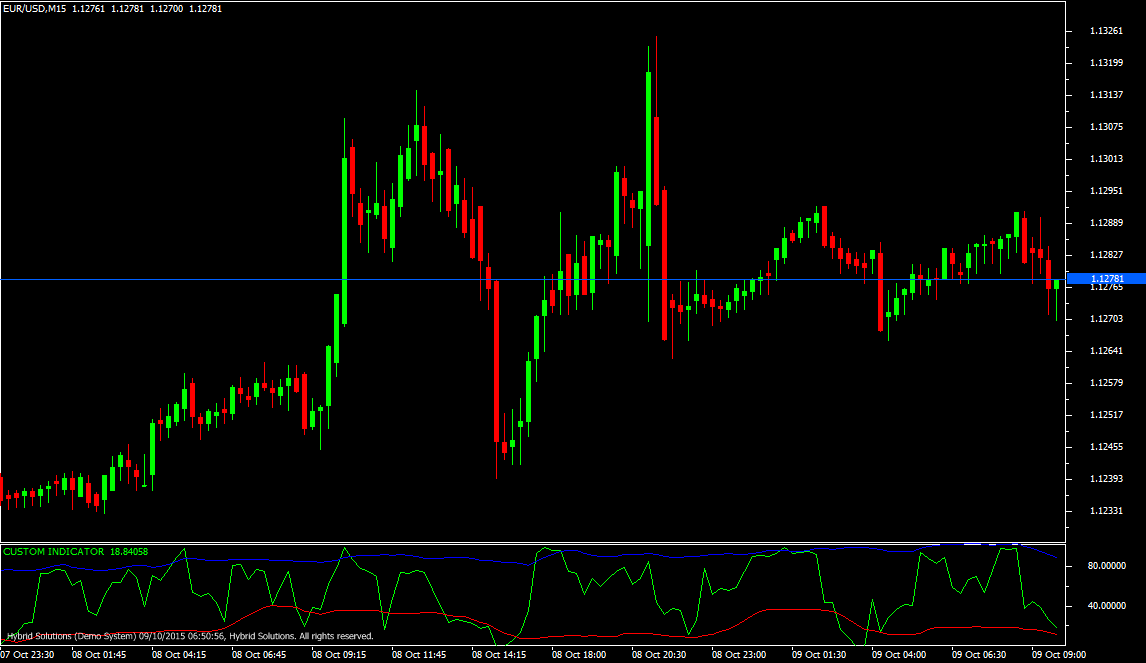# Dynamic Zone RSI

Free

Developed By: NeeduVerdeSolution

In stock

Dynamic Zone RSI, is a VertexFX indicator that calculates the upper and lower zones of the RSI dynamically to determine the trading levels. In standard RSI trading system, the upper and lower trading levels are set to 70 and 30 respectively.

It is advised to sell when RSI falls below 70, and to buy when RSI rises above 30. In Dynamic Zone RSI, we first calculate the standard RSI.

SKU: 726 Categories: ,Dynamic Zone RSI, is a VertexFX indicator that calculates the upper and lower zones of the RSI dynamically to determine the trading levels. In standard RSI trading system, the upper and lower trading levels are set to 70 and 30 respectively.

It is advised to sell when RSI falls below 70, and to buy when RSI rises above 30. In Dynamic Zone RSI, we first calculate the standard RSI. The upper and lower trading zones are calculated based upon standard deviation of the moving average of RSI over BANDS_PERIOD. The upper zone is set to the average RSI plus 1.3185 times the standard deviation of the RSI. The lower zone is set to the average RSI minus 1.3185 times the standard deviation.

The advantage of this is that Dynamic Zone RSI adapts market volatility and provides better trading performance.

BUY – Place Buy position when Dynamic Zone RSI closes above the lower (RED) band from below.
SELL – Place Sell Position when Dynamic Zone RSI closes below the upper (BLUE) band from above.